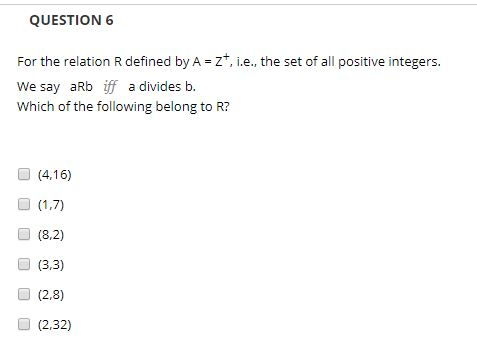# QUESTIONFor the relation R defined by A zt, i.e.., the set of all positive integers.We say aRb iff a divides b.which of the following belong to R?(4,16)(1,7)(8,2)(3,3)(2,8)(2,32)

Question
1 viewshelp_outlineImage TranscriptioncloseQUESTION For the relation R defined by A zt, i.e.., the set of all positive integers. We say aRb iff a divides b. which of the following belong to R? (4,16) (1,7) (8,2) (3,3) (2,8) (2,32) fullscreen
check_circle

star
star
star
star
star
1 Rating
Step 1

Consider the provided question,

Given aRb if a divides b.

And, R defined by A = Z+ that means set o...

### Want to see the full answer?

See Solution

#### Want to see this answer and more?

Solutions are written by subject experts who are available 24/7. Questions are typically answered within 1 hour.*

See Solution
*Response times may vary by subject and question.
Tagged in

### Math